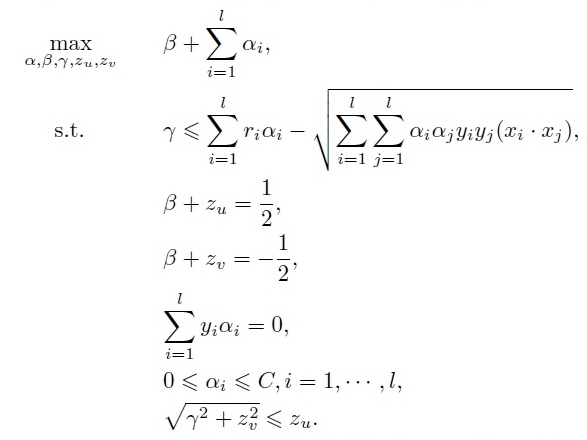# How can I solve this SOCP problem using MOSEK

I would like to solve the following optimization problem using MOSEK. It is known as the Second Order Cone Programming. Without the first constraint, solving this problem by MOSEK is easy, but by adding the first constraint, which includes 2 multiplied variables (αi × αj), I can’t solve it because I did not find a section in the MOSEK help about solving problems with nonlinear constraints including multiplied variables. Please guide me about how I can express the first constraint.Wonder why you ask the question and not in